IF YOU CAN SEE THIS go to /sfa/Nuprl/Shared/Xindentation_hack_doc.html

### Lists

"A List" is a type of finite sequences of members of type A, employing the conventional list constructions from programming.

For a type A,

a.as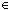A List is the list whose head is aA, and whose tail is asA List.

nilA List is the empty list.

Of course one often sees iterated list expressions, which we display like

[1+2; 0; 4-2]

If the last tail-nested expression is not the constant nil it is displayed like

[1+2; 0; 4-2/ f(x)]

Here is a destructor for lists:

Case of X; nil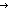e ; x.yf(x,y)

It combines deciding emptiness with list splitting.

Case of nil; nile ; x.yf(x,y) *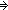e

Case of a.as; nile ; x.yf(x,y) *f(a,as)

There are (derived) operations for treating lists as finite functions, namely a selector "xs[i]" and a list maker "f{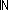n}" from functions.

Thm*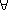n:f:(n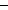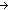T), i:n. (f{n})[i] = f(i)

Thm*as:T List. (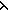i.as[i]){||as||} = as

where ||as|| is list length.

Note on primitive recursion over lists.

There is a primitive, but little used, operation for doing recursion on lists. It is not much used because we tend to use more general methods for defining Recursive Functions but this specific recursion operator has some historical and pedagogical value. Also, in some other type systems, general recursion is not expressible, and such structural recursion operations must be built in.

Case of X; nilb ; x.y, rec:vf(x;y;v) is the form of list recursion.

Case of nil; nilb ; x.y, rec:vf(x;y;v) *b

Case of a.as; nilb ; x.y, rec:vf(x;y;v)
*f(a;as;Case of as; nilb ; x.y, rec:vf(x;y;v))

Notice that there is a third argument to the list-splitting case; the application of the recursive function on the tail is passed in through it.
Actually, our (Case of X; nile ; x.yf(x,y)) operator is simply the special case of this recursion operator in which the recursion on the tail is ignored.

(Sept 2001 - sfa )